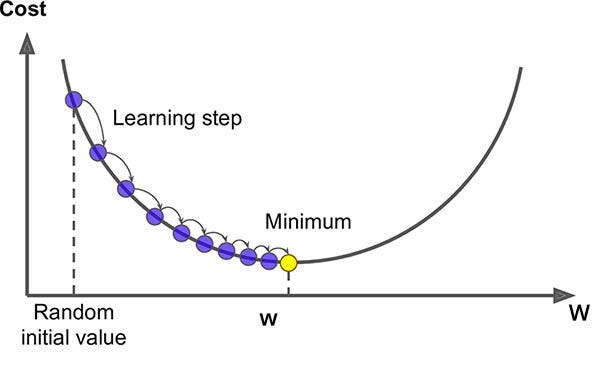# An Introduction to Gradient Descent

Original article was published by Charis Sng on Deep Learning on Medium# An Introduction to Gradient Descent

Gradient Descent is an optimization algorithm used to tweak parameters iteratively to minimize the cost function.

Suppose you’re blindfolded in the mountains, and your goal is to reach the bottom of the valley swiftly.

There are many solutions to reach your goal. One of the good strategy is to go downhill in the direction of the steepest slope.

This is what Gradient Descent does. It measures the local gradient of the error function with regard to the parameter, and it goes in the direction of descending gradient. It reached a minimum once the gradient is zero.

## Learning Rate

The learning rate hyperparameter is an important parameter in Gradient Descent, which is the size of the steps.

If the learning rate is too high, it the algorithm might jump across the valley and possibly even higher than before. This might result in the algorithm diverging, failing to find a good solutions.

If the learning rate is too low, the algorithm will have to go through many iterations to converge, which will take a long time.

## Feature Scaling

When using Gradient Descent, you should ensure that all features have a similar scale.

Cost function has the shape of a bowl. However, if the features have different scales, it will result in an elongated bowl.

For the Gradient Descent on a training set where features have the same scale, the algorithm goes straight toward the minimum, therefore reaching it quickly.

However, for the Gradient Descent on a training set where features does not have the same scale, the algorithm will first goes in a direction almost orthogonal to the direction of the minimum. It will then gradually march down an almost flat valley. It will take a long time before it reach the minimum.

## Types of Gradient Descent Algorithms

Batch Gradient Descent is a variation of the Gradient Descent algorithm that calculates the error for each example in the training dataset, but only updates the model after all training examples have been evaluated.

Since it uses the whole batch of training data to compute the gradient at every step, it result in an extremely slow computation.

Stochastic Gradient Descent algorithm picks a random instance in the training data at every step and computes the gradient based on that single instance. It results in a faster computation than the Batch Gradient Descent.

On the other hand, due to its stochastic nature, Stochastic Gradient Descent is less regular than Batch Gradient Descent. Instead of gently decreasing until it reaches the minimum, the cost function will jump around. Even when it end up close to the minimum, it will still continue to jump around and never settling down at the minimum.

One solution to end this dilemma is to gradually decrease the learning rate. The steps will start out large, and gradually get slower, eventually settling at the minimum.